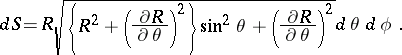# Spherical coordinates

(diff) ← Older revision | Latest revision (diff) | Newer revision → (diff)

The numberswhich are related to the Cartesian coordinates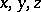by the formulaswhere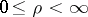,,.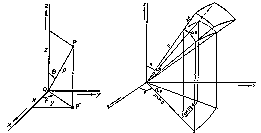Figure: s086660a

The coordinate surfaces are (see Fig.): concentric spheres with centre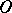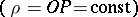; half-planes that pass through the axis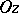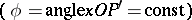; circular cones with vertexand axis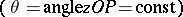. The system of spherical coordinates is orthogonal.

The Lamé coefficients are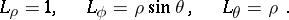The element of surface area is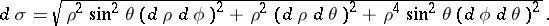The volume element is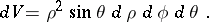The basic operations of vector calculus are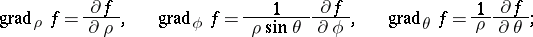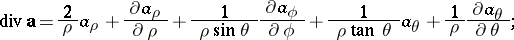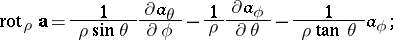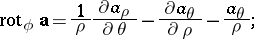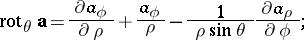The numbers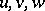, called generalized spherical coordinates, are related to the Cartesian coordinatesby the formulas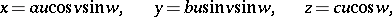where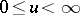,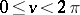,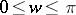,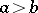,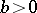. The coordinate surface are: ellipsoids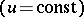, half-planes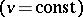and elliptical cones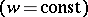.

If the surface has been given by, then the element of surface area can be written as: